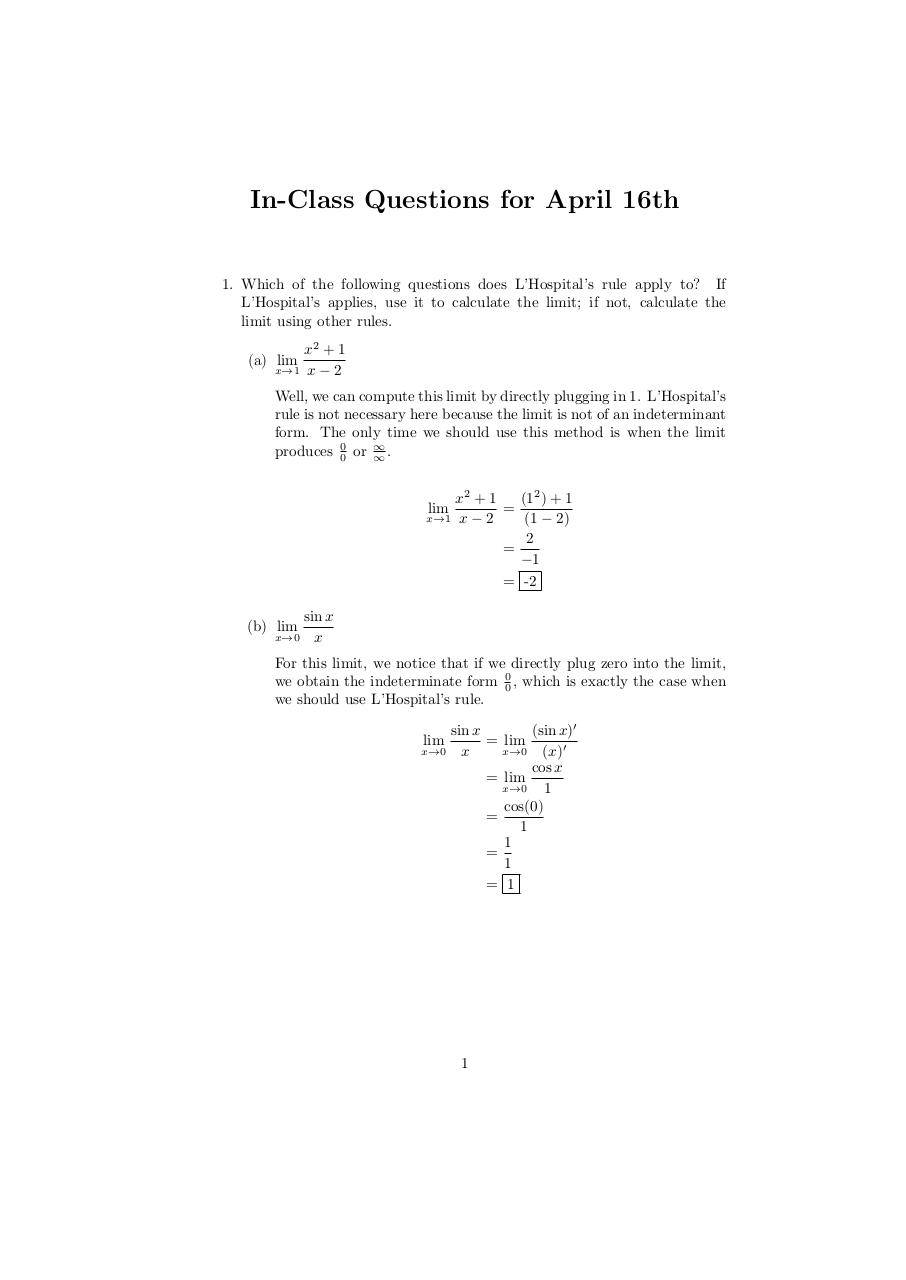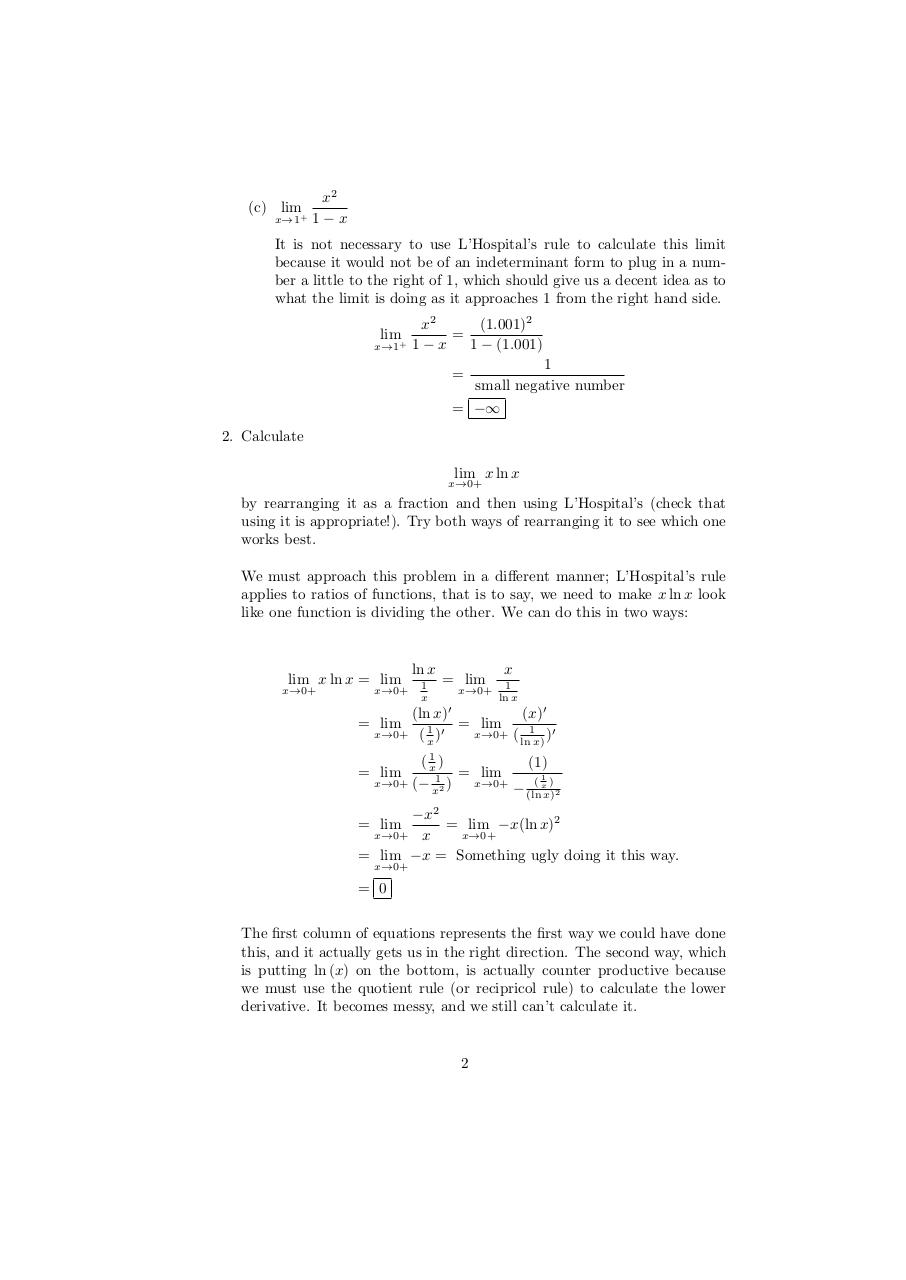# April 16 Solutions .pdf

### File information

Original filename: April 16 Solutions.pdf

This PDF 1.4 document has been generated by TeX output 2012.04.16:2103 / MiKTeX-dvipdfmx (20090708 svn texlive 14695), and has been sent on pdf-archive.com on 17/04/2012 at 02:04, from IP address 198.213.x.x. The current document download page has been viewed 827 times.
File size: 22 KB (2 pages).
Privacy: public file

April 16 Solutions.pdf (PDF, 22 KB)

### Document preview

In-Class Questions for April 16th

1. Which of the following questions does L’Hospital’s rule apply to? If
L’Hospital’s applies, use it to calculate the limit; if not, calculate the
limit using other rules.
x2 + 1
x→1 x − 2

(a) lim

Well, we can compute this limit by directly plugging in 1. L’Hospital’s
rule is not necessary here because the limit is not of an indeterminant
form. The only time we should use this method is when the limit
produces 00 or ∞
∞.
(12 ) + 1
x2 + 1
=
x→1 x − 2
(1 − 2)
2
=
−1
= -2
lim

(b) lim

x→0

sin x
x

For this limit, we notice that if we directly plug zero into the limit,
we obtain the indeterminate form 00 , which is exactly the case when
we should use L’Hospital’s rule.
sin x
(sin x)′
= lim
x→0 x
x→0 (x)′
cos x
= lim
x→0
1
cos(0)
=
1
1
=
1
= 1
lim

1

(c) lim+
x→1

x2
1−x

It is not necessary to use L’Hospital’s rule to calculate this limit
because it would not be of an indeterminant form to plug in a number a little to the right of 1, which should give us a decent idea as to
what the limit is doing as it approaches 1 from the right hand side.
lim+

x→1

(1.001)2
x2
=
1−x
1 − (1.001)
=

1
small negative number

= −∞
2. Calculate
lim x ln x

x→0+

by rearranging it as a fraction and then using L’Hospital’s (check that
using it is appropriate!). Try both ways of rearranging it to see which one
works best.
We must approach this problem in a diﬀerent manner; L’Hospital’s rule
applies to ratios of functions, that is to say, we need to make x ln x look
like one function is dividing the other. We can do this in two ways:

lim x ln x = lim

x→0+

x→0+

ln x
1
x

= lim

x→0+

x
1
ln x

(ln x)′
(x)′
= lim
1

x→0+ ( )
x→0+ ( 1 )′
x
ln x)

= lim

( x1 )
(1)
= lim
(1)
x→0+ (− 12 )
x→0+
− x 2
x

= lim

(ln x)

−x2
= lim
= lim −x(ln x)2
x→0+ x
x→0+
= lim −x = Something ugly doing it this way.
x→0+

= 0
The ﬁrst column of equations represents the ﬁrst way we could have done
this, and it actually gets us in the right direction. The second way, which
is putting ln (x) on the bottom, is actually counter productive because
we must use the quotient rule (or recipricol rule) to calculate the lower
derivative. It becomes messy, and we still can’t calculate it.

2#### HTML Code

Copy the following HTML code to share your document on a Website or Blog

#### QR Code### Related keywords